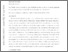# Discrete convolution statistic for hypothesis testing

Prevedello, Giulio and Duffy, Ken R. (2020) Discrete convolution statistic for hypothesis testing. Communications in Statistics - Theory and Methods. ISSN 1532-415XPreviewmore...Add this article to your Mendeley library

## Abstract

The question of testing for equality in distribution between two linear models, each consisting of sums of distinct discrete independent random variables with unequal numbers of observations, has emerged from the biological research. In this case, the computation of classical χ2 statistics, which would not include all observations, results in loss of power, especially when sample sizes are small. Here, as an alternative that uses all data, the maximum likelihood estimator for the distribution of sum of discrete and independent random variables, which we call the convolution statistic, is proposed and its limiting normal covariance matrix determined. To challenge null hypotheses about the distribution of this sum, the generalized Wald’s method is applied to define a testing statistic whose distribution is asymptotic to a χ2 with as many degrees of freedom as the rank of such covariance matrix. Rank analysis also reveals a connection with the roots of the probability generating functions associated to the addend variables of the linear models. A simulation study is performed to compare the convolution test with Pearson’s χ2, and to provide usage guidelines.

Item Type: Article iscrete convolution; sum of discrete random variables; statistical hypothesis testing; nonparametric maximum-likelihood estimation; sub-independence; Faculty of Science and Engineering > Research Institutes > Hamilton Institute 15201 https://doi.org/10.1080/03610926.2020.1811336 Dr Ken Duffy 10 Jan 2022 12:05 Communications in Statistics - Theory and Methods Taylor & Francis YesItem control page